## Natural Logarithm

The Logarithm having base e, where(1)

which can be defined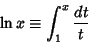(2)

for. The natural logarithm can also be defined for Complex Numbers as(3)

where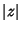is the Modulus andis the Argument. The natural logarithm is especially useful in Calculus because its Derivative is given by the simple equation(4)

whereas logarithms in other bases have the more complicated Derivative(5)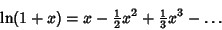(6)

gives a Taylor Series for the natural logarithm.

Continued Fraction representations of logarithmic functions include(7)(8)

For a Complex Number, the natural logarithm satisfies(9)(10)

whereis the Principal Value.

Some special values of the natural logarithm are(11)(12)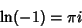(13)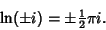(14)

An identity for the natural logarithm of 2 discovered using the PSLQ Algorithm is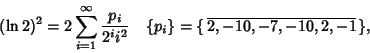(15)

whereis given by the periodic sequence obtained by appending copies of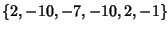(in other words,for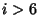) (Bailey et al. 1995, Bailey and Plouffe).

Bailey, D.; Borwein, P.; and Plouffe, S. On the Rapid Computation of Various Polylogarithmic Constants.'' http://www.cecm.sfu.ca/~pborwein/PAPERS/P123.ps.
Bailey, D. and Plouffe, S. Recognizing Numerical Constants.'' http://www.cecm.sfu.ca/organics/papers/bailey/.